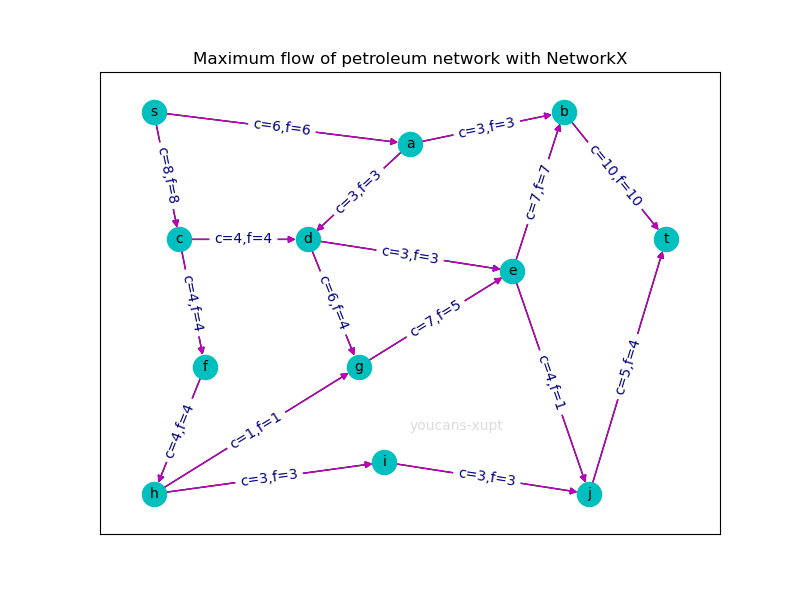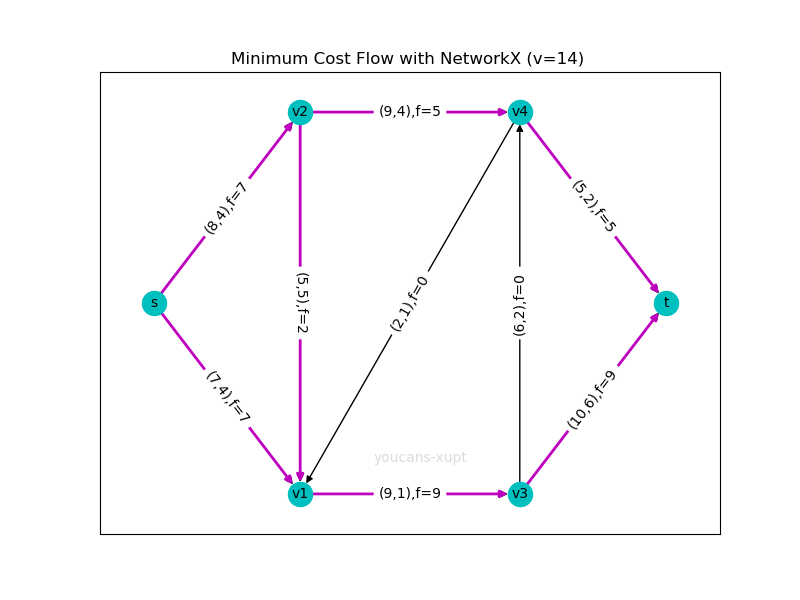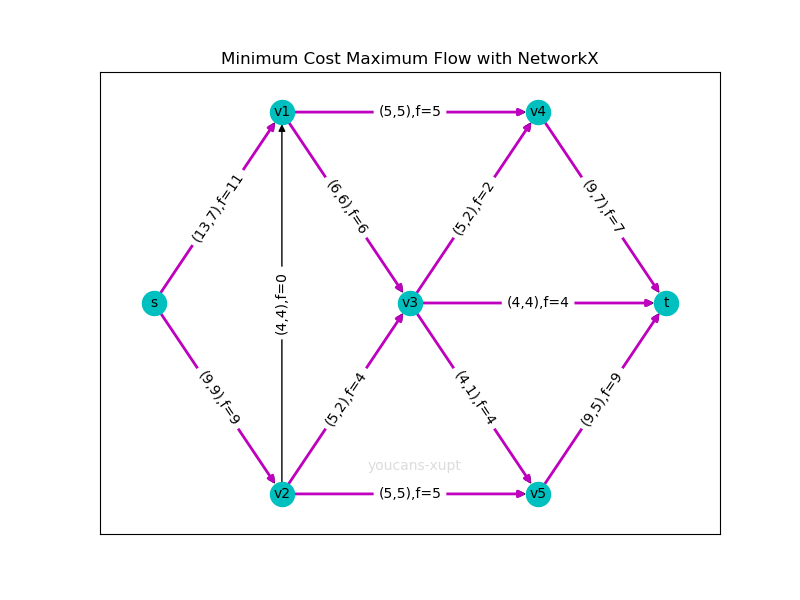# Python小白的数学建模课-19.网络流优化问题

• 流在生活中十分常见，例如交通系统中的人流、车流、物流，供水管网中的水流，金融系统中的现金流，网络中的信息流。网络流优化问题是基本的网络优化问题，应用非常广泛。
• 网络流优化问题最重要的指标是边的成本和容量限制，既要考虑成本最低，又要满足容量限制，由此产生了网络最大流问题、最小费用流问题、最小费用最大流问题。
• 本文基于 NetworkX 工具包，通过例程详细介绍网络最大流问题、最小费用流问题、最小费用最大流问题的建模和编程。
• 『Python小白的数学建模课 @ Youcans』带你从数模小白成为国赛达人。

## 1. 网络流优化

### 1.1 网络流

• 源点/汇点，起点/终点，供应点/需求点：源点是只进不出的点，汇点是只出不进的点。源点/汇点 可以指定为问题的 起点/终点，但本质上源点/汇点是由网络结构特征决定的，而不是被指定的。供应点的供应量和需求点的需求量是固定/确定的，而源点/汇点的目标是发出/接收的流量最大，不是固定值。
• 容量 与 流量：容量是路径（边、弧）允许的最大流通能力，用 c(i,j) 表示；流量则是路径（边、弧）上的实际流量，用 f(i,j) 表示。

### 1.2 典型的网络流优化问题

Network 工具包求解网络流优化，包括最大流算法、最小割算法、最小费用流算法、最小费用最大流算法、容量缩放最小成本流算法。

## 2. 网络最大流问题（MFP）

### 2.2 NetworkX 求解网络最大流问题

Network 工具包提供了多种求解网络最大流问题的算法和函数。其中 maximum_flow()、maximum_flow_value()、minimum_cut()、minimum_cut_value() 是集成了多种算法的通用函数，可以设置算法选项调用对应的算法；其它函数则是具体的算法实现函数。

maximum_flow(flowG,s,t[, capacity,...]) 计算最大流
maximum_flow_value(flowG,s,t[,...]) 计算最大的单一目标流的值
minimum_cut(flowG,s,t[, capacity,flow_func]) 计算最小割的值和节点分区
minimum_cut_value(flowG,s,t[,capacity,...]) 计算最小割的值
edmonds_karp(G,s,t[,capacity,...]) Edmonds-Karp 算法求最大流
shortest_augmenting_path(G,s,t[,...]) SAP算法求最大流
dinitz(G,s,t[,capacity,...]) Dinitz 算法求最大流
preflow_push(G,s,t[,capacity,...]) HLPP 算法求最大流
boykov_kolmogorov(G,s,t[,capacity,...]) Boykov-Kolmogorov 算法求最大流

### 2.3 maximum_flow() 函数说明

maximum_flow()maximum_flow_value() 是求解网络最大流问题的通用方法，集成了多种算法可供选择。官网介绍详见：https://networkx.org/documentation/stable/reference/algorithms/flow.html

maximum_flow (flowG, _s, _t, capacity='capacity', flow_func=None, *kwargs)
maximum_flow_value (flowG, _s, _t, capacity='capacity', flow_func=None, *kwargs)

• flowG(NetworkX graph)：有向图，边必须带有容量属性 capacity（不能用 'weight' ）。
• _s (node)：源点。
• _t (node)：汇点。
• capacity (string)：边的容量属性 capacity，缺省视为无限容量。
• flow_func(function)：调用算法的函数名，如：'edmonds_karp', 'shortest_augmenting_path', 'dinitz', 'preflow_push', 'boykov_kolmogorov'。缺省值 'None' ，选择 'preflow_push'（HLPP 算法）。

• flow_value(integer, float)：网络最大流的流量值
• flow_dict (dict)：字典类型，网络最大流的流经路径及各路径的分配流量

from networkx.algorithms.flow import edmonds_karp
flowValue, flowDict = nx.maximum_flow(G1, 's', 't', flow_func=edmonds_karp)


### 2.4 案例：输油管网的最大流量

1. 图的输入。本例为稀疏有向图，使用 nx.DiGraph() 定义一个有向图。通过 add_edge('s', 'a', capacity=6) 定义有向边和属性 capacity。注意必须以关键字 'capacity' 表示容量，不能用权值 'weight' 或其它关键字代替。

2. nx.maximum_flow_value() 返回网络最大流的值，nx.maximum_flow() 可以同时返回网络最大流的值和网络最大流的路径及分配的流量。

3. maxFlowDict 为字典类型，具体格式参加 2.6 程序运行结果。为了得到最大流所流经的边的列表edgeLists，要对 maxFlowDict 进行整理和格式转换。

4. 在网络最大流图中，以边的标签显示了边的容量 c 和流量 f。

### 2.5 Python 例程

# mathmodel19_v1.py
# Demo19 of mathematical modeling algorithm
# Demo of network flow problem optimization with NetworkX
# Crated：2021-07-15

import numpy as np
import matplotlib.pyplot as plt # 导入 Matplotlib 工具包
import networkx as nx  # 导入 NetworkX 工具包

# 1. 最大流问题 (Maximum Flow Problem，MFP)
# 创建有向图
G1 = nx.DiGraph()  # 创建一个有向图 DiGraph
G1.add_edge('s', 'a', capacity=6)  # 添加边的属性 "capacity"

# 求网络最大流
# maxFlowValue = nx.maximum_flow_value(G1, 's', 't')  # 求网络最大流的值
# maxFlowValue, maxFlowDict = nx.maximum_flow(G1, 's', 't')  # 求网络最大流
from networkx.algorithms.flow import edmonds_karp  # 导入 edmonds_karp 算法函数
maxFlowValue, maxFlowDict = nx.maximum_flow(G1, 's', 't', flow_func=edmonds_karp)  # 求网络最大流

# 数据格式转换
edgeCapacity = nx.get_edge_attributes(G1, 'capacity')
edgeLabel = {}  # 边的标签
for i in edgeCapacity.keys():  # 整理边的标签，用于绘图显示
edgeLabel[i] = f'c={edgeCapacity[i]:}'  # 边的容量
edgeLists = []  # 最大流的边的 list
for i in maxFlowDict.keys():
for j in maxFlowDict[i].keys():
edgeLabel[(i, j)] += ',f=' + str(maxFlowDict[i][j])  # 取出每条边流量信息存入边显示值
if maxFlowDict[i][j] > 0:  # 网络最大流的边（流量>0）
edgeLists.append((i,j))

# 输出显示
print("最大流值: ", maxFlowValue)
print("最大流的途径及流量: ", maxFlowDict)  # 输出最大流的途径和各路径上的流量
print("最大流的路径：", edgeLists)  # 输出最大流的途径

# 绘制有向网络图
fig, ax = plt.subplots(figsize=(8, 6))
pos = {'s': (1, 8), 'a': (6, 7.5), 'b': (9, 8), 'c': (1.5, 6), 'd': (4, 6), 'e': (8, 5.5),  # 指定顶点绘图位置
'f': (2, 4), 'g': (5, 4), 'h': (1, 2), 'i': (5.5, 2.5), 'j': (9.5, 2), 't': (11, 6)}
edge_labels = nx.get_edge_attributes(G1, 'capacity')
ax.set_title("Maximum flow of petroleum network with NetworkX")  # 设置标题
nx.draw(G1, pos, with_labels=True, node_color='c', node_size=300, font_size=10)  # 绘制有向图，显示顶点标签
nx.draw_networkx_edge_labels(G1, pos, edgeLabel, font_color='navy')  # 显示边的标签：'capacity' + maxFlow
nx.draw_networkx_edges(G1, pos, edgelist=edgeLists, edge_color='m')  # 设置指定边的颜色、宽度
plt.axis('on')  # Youcans@XUPT
plt.show()


### 2.6 程序运行结果

最大流值:  14## 3. 最小费用流问题（MCP）

### 3.1 最小费用流问题的算法

\begin{align*} & min\;Cost=\sum_{i=1}^m\sum_{j=1}^m w_{ij} f_{ij}\\ & s.t.:\;\begin{cases} \sum_{j=1}^m f_{ij} - \sum_{j=1}^m f_{ji} = v_i\\ \sum_{i=1}^m v_{i} = 0\\ 0 \leq f_{ij} \leq c_{ij} \; \end{cases} \end{align*}

### 3.2 NetworkX 求解最小费用流问题

Network 工具包提供了多个求解最小费用流问题的函数，所用的基本算法都是网络单纯性算法。

network_simplex(G,[,demand,capacity,weight]) 单纯性法计算最小成本流
min_cost_flow_cost(G,[,demand,capacity,weight]) 计算最小成本流的成本
min_cost_flow(G,[,demand,capacity,weight]) 计算最小成本流
max_flow_min_cost(G,s,t[,capacity,weight]) 计算最小成本的最大流
capacity_scaling(G[,demand,capacity,...]) 计算容量缩放最小成本流

### 3.3 min_cost_flow() 函数说明

min_cost_flow()min_cost_flow_cost() 是求解费用最小流问题的函数，通过调用网络单纯性算法函数 network_simplex() 求解。

min_cost_flow(G, demand='demand', capacity='capacity', weight='weight')
min_cost_flow_cost(G, demand='demand', capacity='capacity', weight='weight')

• G(NetworkX graph)：有向图，边必须带有容量属性 capacity、单位成本属性 'weight' 。
• demand (string)：顶点的需求量属性 demand，表示节点的净流量：负数表示供应点的净流出量，正数表示需求点的净流入量，0 表示中转节点。缺省值为 0。
• capacity (string)：边的容量，缺省视为无限容量。
• weight (string)：边的单位流量的费用，缺省值为 0。

• flowDict (dict)：字典类型，最小费用流的流经路径及各路径的分配流量
• flowCost(integer, float)：满足需求的最小费用流的总费用
• NetworkXUnfeasible：输入的净流量(demand)不平衡，或没有满足需求流量的可行流时，抛出异常信息。

### 3.4 案例：运输费用

1. 图的输入。本例为稀疏的有向图，使用 nx.DiGraph() 定义一个有向图，用G.add_weighted_edges_from() 函数以列表向图中添加多条赋权边，每个赋权边以元组 (node1,node2,{'capacity':c1, 'weight':w1}) 定义属性 'capacity' 和 'weight'。注意必须以关键字 'capacity' 表示容量，以 'weight' 表示单位流量的费用。
2. nx.shortest_path() 用于计算最短路径，该段不是必须的。将最短路径的计算结果与最小费用流的结果进行比较，可以看到流量 v=1 时最小费用流的结果与最短路径结果是相同的。
3. nx.cost_of_flow() 用于计算最小费用最大流，该段不是必须的。将最小费用最大流的计算结果与最小费用流的结果进行比较，可以看到在最大流量 v=14 时最小费用流的结果与最小费用最大流结果是相同的。
4. 最小费用流是基于确定的流量 v 而言的。流量 v 可以在程序中赋值；例程中 v 从 1 逐渐递增，计算所有流量下的最小费用流，直到达到网络容量的极限（如果再增大流量将会超出网络最大容量，没有可行流，计算最小费用流失败）。
5. NetworkX 计算最小费用流时不是在函数中指定源点、汇点和流量，而是通过向源点、汇点添加属性 demand 实现的。demand 为正值时表示净输入流量，demand 为负值时表示净输出流量，这使我们可以指定多源多汇。
6. nx.min_cost_flow() 返回最小费用流的路径和流量分配，字典格式；nx.min_cost_flow_cost() 返回最小费用流的费用值。nx.network_simplex() 也可以求最小费用流，返回最小费用流的费用值，路径和流量分配。
7. 在最小费用流图中（最大流量 v=14），以边的标签显示了边的容量 c、单位流量的费用 w 和流量 f，如 (8,4),f=7 表示边的容量为 8，单位流量的费用为 4，分配流量为 7。
8. 在最小费用流图中（最大流量 v=14），以不同颜色（edge_color='m'）和宽度（width=2）表示最小费用流的边，未使用的流量为 0 （f=0）的边以黑色绘制。

### 3.5 Python 例程：

# mathmodel19_v1.py
# Demo19 of mathematical modeling algorithm
# Demo of network flow problem optimization with NetworkX
# Crated：2021-07-15

import numpy as np
import matplotlib.pyplot as plt # 导入 Matplotlib 工具包
import networkx as nx  # 导入 NetworkX 工具包

# 2. 最小费用流问题（Minimum Cost Flow，MCF）
# 创建有向图
G2 = nx.DiGraph()  # 创建一个有向图 DiGraph
('s','v2',{'capacity': 8, 'weight': 4}),
('v1','v3',{'capacity': 9, 'weight': 1}),
('v2','v1',{'capacity': 5, 'weight': 5}),
('v2','v4',{'capacity': 9, 'weight': 4}),
('v3','v4',{'capacity': 6, 'weight': 2}),
('v3','t',{'capacity': 10, 'weight': 6}),
('v4','v1',{'capacity': 2, 'weight': 1}),
('v4','t',{'capacity': 5, 'weight': 2})]) # 添加边的属性 'capacity', 'weight'
# 整理边的标签，用于绘图显示
edgeLabel1 = nx.get_edge_attributes(G2, 'capacity')
edgeLabel2 = nx.get_edge_attributes(G2, 'weight')
edgeLabel = {}
for i in edgeLabel1.keys():
edgeLabel[i] = f'({edgeLabel1[i]:},{edgeLabel2[i]:})'  # 边的(容量，成本)

# 计算最短路径---非必要，用于与最小费用流的结果进行比较
lenShortestPath = nx.shortest_path_length(G2, 's', 't', weight="weight")
shortestPath = nx.shortest_path(G2, 's', 't', weight="weight")
print("\n最短路径: ", shortestPath)  # 输出最短路径
print("最短路径长度: ", lenShortestPath)  # 输出最短路径长度

# 计算最小费用最大流---非必要，用于与最小费用流的结果进行比较
minCostFlow = nx.max_flow_min_cost(G2, 's', 't')  # 求最小费用最大流
minCost = nx.cost_of_flow(G2, minCostFlow)  # 求最小费用的值
maxFlow = sum(minCostFlow['s'][j] for j in minCostFlow['s'].keys())  # 求最大流量的值
print("\n最大流量: {}".format(maxFlow))  # 输出最小费用的值
print("最大流量的最小费用: {}\n".format(minCost))  # 输出最小费用的值

# v = input("Input flow (v>=0):")
v = 0
while True:
v += 1  # 最小费用流的流量

try: # Youcans@XUPT
# 求最小费用流(demand=v)
minFlowCost = nx.min_cost_flow_cost(G2)  # 求最小费用流的费用
minFlowDict = nx.min_cost_flow(G2)  # 求最小费用流
# minFlowCost, minFlowDict = nx.network_simplex(G2)  # 求最小费用流--与上行等效
print("流量: {:2d}\t最小费用:{}".format(v, minFlowCost))  # 输出最小费用的值(demand=v)
# print("最小费用流的路径及流量: ", minFlowDict)  # 输出最大流的途径和各路径上的流量
except Exception as e:
print("流量: {:2d}\t超出网络最大容量，没有可行流。".format(v))
print("\n流量 v={:2d}：计算最小费用流失败({})。".format(v, str(e)))
break  # 结束 while True 循环

edgeLists = []
for i in minFlowDict.keys():
for j in minFlowDict[i].keys():
edgeLabel[(i, j)] += ',f=' + str(minFlowDict[i][j])  # 取出每条边流量信息存入边显示值
if minFlowDict[i][j] > 0:
edgeLists.append((i, j))

maxFlow = sum(minFlowDict['s'][j] for j in minFlowDict['s'].keys())  # 求最大流量的值
print("\n最大流量: {:2d},\t最小费用:{}".format(maxFlow, minFlowCost))  # 输出最小费用的值
print("最小费用流的路径及流量: ", minFlowDict)  # 输出最小费用流的途径和各路径上的流量
print("最小费用流的路径：", edgeLists)  # 输出最小费用流的途径

# 绘制有向网络图
pos={'s':(0,5),'v1':(4,2),'v2':(4,8),'v3':(10,2),'v4':(10,8),'t':(14,5)}  # 指定顶点绘图位置
fig, ax = plt.subplots(figsize=(8,6))
ax.text(6,2.5,"youcans-xupt",color='gainsboro')
ax.set_title("Minimum Cost Maximum Flow with NetworkX")
nx.draw(G2,pos,with_labels=True,node_color='c',node_size=300,font_size=10)   # 绘制有向图，显示顶点标签
nx.draw_networkx_edge_labels(G2,pos,edgeLabel,font_size=10)  # 显示边的标签：'capacity','weight' + minCostFlow
nx.draw_networkx_edges(G2,pos,edgelist=edgeLists,edge_color='m',width=2)  # 设置指定边的颜色、宽度
plt.axis('on')
plt.show()


### 3.6 程序运行结果：

最短路径:  ['s', 'v1', 'v3', 'v4', 't']## 4. 最小费用最大流问题（MCMF）

### 4.2 max_flow_min_cost() 函数说明

max_flow_min_cost()是求解最小费用最大流问题的函数。

max_flow_min_cost(G, s, t, capacity='capacity', weight='weight')
cost_of_flow(G, flowDict, weight='weight')

• G(NetworkX graph)：有向图，边必须带有容量属性 capacity、单位成本属性 'weight' 。
• s (node)：流的源点。
• t (node)：流的汇点。
• capacity (string)：边的容量，缺省视为无限容量。
• weight (string)：边的单位流量的费用，缺省值为 0。

• flowDict (dict)：字典类型，最小费用最大流的流经路径及各路径的分配流量。

### 4.3 案例：输油管网的最大流量和最小费用

1. 图的输入。用 nx.DiGraph() 定义一个有向图。用 G.add_weighted_edges_from() 函数以列表向图中添加多条赋权边，每个赋权边以元组 (node1,node2,{'capacity':c1, 'weight':w1}) 定义属性 'capacity' 和 'weight'。注意必须以关键字 'capacity' 表示容量，以 'weight' 表示单位流量的费用。
2. nx.max_flow_min_cost(G3, 's', 't') 用来计算从源点 's' 到汇点 't' 的最小费用最大流，返回最大流的途径和各路径上的流量分配，字典格式。
3. nx.cost_of_flow() 计算一个可行流的费用，例程中用来计算最小费用最大流的费用。
4. maxFlow 计算从源点 's' 发出的所有路径上的流量总和，得到最大流量的值。
5. 在最小费用最大流图中，以边的标签显示了边的容量 c、单位流量的费用 w 和流量 f，如 (13,7),f=11表示边的容量为 13，单位流量的费用为 7，分配流量为 11。
6. 在最小费用最大流图中，以不同颜色（edge_color='m'）和宽度（width=2）表示最小费用流的边，未使用的流量为 0 （f=0）的边以黑色绘制。

### 4.4 Python 例程

# mathmodel19_v1.py
# Demo19 of mathematical modeling algorithm
# Demo of network flow problem optimization with NetworkX
# Crated：2021-07-15

import numpy as np
import matplotlib.pyplot as plt # 导入 Matplotlib 工具包
import networkx as nx  # 导入 NetworkX 工具包

# 3. 最小费用最大流问题（Minimum Cost Maximum Flow，MCMF）
# 创建有向图
G3 = nx.DiGraph()  # 创建一个有向图 DiGraph
('s','v2',{'capacity': 9, 'weight': 9}),
('v1','v3',{'capacity': 6, 'weight': 6}),
('v1','v4',{'capacity': 5, 'weight': 5}),
('v2','v1',{'capacity': 4, 'weight': 4}),
('v2','v3',{'capacity': 5, 'weight': 2}),
('v2','v5',{'capacity': 5, 'weight': 5}),
('v3','v4',{'capacity': 5, 'weight': 2}),
('v3','v5',{'capacity': 4, 'weight': 1}),
('v3','t',{'capacity': 4, 'weight': 4}),
('v4','t', {'capacity': 9, 'weight': 7}),
('v5','t',{'capacity': 9, 'weight': 5})]) # 添加边的属性 'capacity', 'weight'

# 求最小费用最大流
minCostFlow = nx.max_flow_min_cost(G3, 's', 't')  # 求最小费用最大流
minCost = nx.cost_of_flow(G3, minCostFlow)  # 求最小费用的值
maxFlow = sum(minCostFlow['s'][j] for j in minCostFlow['s'].keys())  # 求最大流量的值

# # 数据格式转换
edgeLabel1 = nx.get_edge_attributes(G3,'capacity')  # 整理边的标签，用于绘图显示
edgeLabel2 = nx.get_edge_attributes(G3,'weight')
edgeLabel={}
for i in edgeLabel1.keys():
edgeLabel[i]=f'({edgeLabel1[i]:},{edgeLabel2[i]:})'  # 边的(容量，成本)
edgeLists = []
for i in minCostFlow.keys():
for j in minCostFlow[i].keys():
edgeLabel[(i, j)] += ',f=' + str(minCostFlow[i][j])  # 将边的实际流量添加到 边的标签
if minCostFlow[i][j]>0:
edgeLists.append((i,j))

print("最小费用最大流的路径及流量: ", minCostFlow)  # 输出最大流的途径和各路径上的流量
print("最小费用最大流的路径：", edgeLists)  # 输出最小费用最大流的途径
print("最大流量: ", maxFlow)  # 输出最大流量的值
print("最小费用: ", minCost)  # 输出最小费用的值

# 绘制有向网络图
pos={'s':(0,5), 'v1':(3,9), 'v2':(3,1), 'v3':(6,5), 'v4':(9,9),'v5':(9,1), 't':(12,5)}  # 指定顶点绘图位置
fig, ax = plt.subplots(figsize=(8,6))
ax.text(5,1.5,"youcans-xupt",color='gainsboro')
ax.set_title("Minimum Cost Maximum Flow with NetworkX")
nx.draw(G3,pos,with_labels=True,node_color='c',node_size=300,font_size=10)   # 绘制有向图，显示顶点标签
nx.draw_networkx_edge_labels(G3,pos,edgeLabel,font_size=10)  # 显示边的标签：'capacity','weight' + minCostFlow
nx.draw_networkx_edges(G3,pos,edgelist=edgeLists,edge_color='m',width=2)  # 设置指定边的颜色、宽度
plt.axis('on') # Youcans@XUPT
plt.show()


### 4.5 运行结果

最小费用最大流的路径及流量:  {'s': {'v1': 11, 'v2': 9}, 'v1': {'v3': 6, 'v4': 5}, 'v2': {'v1': 0, 'v3': 4, 'v5': 5}, 'v3': {'v4': 2, 'v5': 4, 't': 4}, 'v4': {'t': 7}, 'v5': {'t': 9}, 't': {}}## 5. 总结

1. 本文基于 NetworkX 工具包，通过例程详细介绍了网络最大流问题、最小费用流问题、最小费用最大流问题的建模和编程。
2. 运输问题、指派问题、转运问题、最大流问题、最短路径问题，都是特殊情况下的最小费用流问题。通过 3.6 中最短路径、最小费用最大流的结果与 v=1、v=14 的最小费用流结果的比较，可以理解这种关系。
3. 例程给出了对部分指定的边设置颜色，为边设置指定格式的显示内容，NetworkX 函数输出值的数据格式转换的编程方法，建议读者多加留意。
4. 网络流优化问题还有很多变形和衍生问题，将在今后的文中进行介绍。

【本节完】